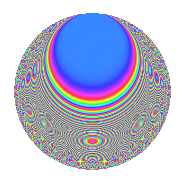# Properties

 Label 403.2.iLevel 403 Weight 2 Character orbit i Rep. character $$\chi_{403}(216,\cdot)$$ Character field $$\Q(\zeta_{4})$$ Dimension 68 Newform subspaces 1 Sturm bound 74 Trace bound 0

# Related objects

## Defining parameters

 Level: $$N$$ = $$403 = 13 \cdot 31$$ Weight: $$k$$ = $$2$$ Character orbit: $$[\chi]$$ = 403.i (of order $$4$$ and degree $$2$$) Character conductor: $$\operatorname{cond}(\chi)$$ = $$403$$ Character field: $$\Q(i)$$ Newform subspaces: $$1$$ Sturm bound: $$74$$ Trace bound: $$0$$

## Dimensions

The following table gives the dimensions of various subspaces of $$M_{2}(403, [\chi])$$.

Total New Old
Modular forms 76 76 0
Cusp forms 68 68 0
Eisenstein series 8 8 0

## Trace form

 $$68q - 4q^{2} - 4q^{5} + 8q^{7} + 16q^{8} - 60q^{9} + O(q^{10})$$ $$68q - 4q^{2} - 4q^{5} + 8q^{7} + 16q^{8} - 60q^{9} - 48q^{14} - 40q^{16} + 4q^{18} - 24q^{19} - 16q^{20} + 44q^{28} + 24q^{31} + 28q^{32} - 40q^{35} - 24q^{39} + 24q^{40} + 20q^{41} - 24q^{45} - 36q^{47} + 80q^{50} + 28q^{59} - 76q^{63} + 152q^{66} - 32q^{67} - 48q^{70} + 20q^{71} - 32q^{72} + 72q^{76} + 84q^{78} - 20q^{80} + 52q^{81} - 112q^{87} - 8q^{93} - 16q^{94} - 4q^{97} - 92q^{98} + O(q^{100})$$

## Decomposition of $$S_{2}^{\mathrm{new}}(403, [\chi])$$ into newform subspaces

Label Dim. $$A$$ Field CM Traces $q$-expansion
$$a_2$$ $$a_3$$ $$a_5$$ $$a_7$$
403.2.i.a $$68$$ $$3.218$$ None $$-4$$ $$0$$ $$-4$$ $$8$$

## Hecke Characteristic Polynomials

There are no characteristic polynomials of Hecke operators in the database